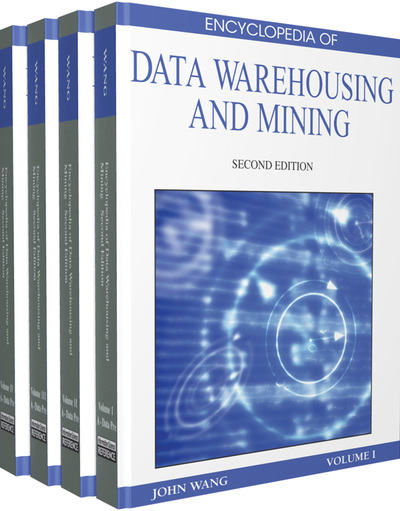# Cluster Analysis in Fitting Mixtures of Curves

Tom Burr (Los Alamos National Laboratory, USA)
DOI: 10.4018/978-1-60566-010-3.ch036
Available
\$37.50
No Current Special Offers

## Abstract

One data mining activity is cluster analysis, which consists of segregating study units into relatively homogeneous groups. There are several types of cluster analysis; one type deserving special attention is clustering that arises due to a mixture of curves. A mixture distribution is a combination of two or more distributions. For example, a bimodal distribution could be a mix with 30% of the values generated from one unimodal distribution and 70% of the values generated from a second unimodal distribution. The special type of mixture we consider here is a mixture of curves in a two-dimensional scatter plot. Imagine a collection of hundreds or thousands of scatter plots, each containing a few hundred points including background noise but also containing from zero to four or five bands of points, each having a curved shape. In one application (Burr et al. 2001), each curved band of points was a potential thunderstorm event (see Figure 1) as observed from a distant satellite and the goal was to cluster the points into groups associated with thunderstorm events. Each curve has its own shape, length, and location, with varying degrees of curve overlap, point density, and noise magnitude. The scatter plots of points from curves having small noise resemble a smooth curve with very little vertical variation from the curve, but there can be a wide range in noise magnitude so that some events have large vertical variation from the center of the band. In this context, each curve is a cluster and the challenge is to use only the observations to estimate how many curves comprise the mixture, plus their shapes and locations. To achieve that goal, the human eye could train a classifier by providing cluster labels to all points in example scatter plots. Each point would either belong to a curved-region or to a catch-all noise category and a specialized cluster analysis would be used to develop an approach for labeling (clustering) the points generated according to the same mechanism in future scatter plots.
Chapter Preview
Top

## Introduction

One data mining activity is cluster analysis, which consists of segregating study units into relatively homogeneous groups. There are several types of cluster analysis; one type deserving special attention is clustering that arises due to a mixture of curves. A mixture distribution is a combination of two or more distributions. For example, a bimodal distribution could be a mix with 30% of the values generated from one unimodal distribution and 70% of the values generated from a second unimodal distribution.

The special type of mixture we consider here is a mixture of curves in a two-dimensional scatter plot. Imagine a collection of hundreds or thousands of scatter plots, each containing a few hundred points including background noise but also containing from zero to four or five bands of points, each having a curved shape. In one application (Burr et al. 2001), each curved band of points was a potential thunderstorm event (see Figure 1) as observed from a distant satellite and the goal was to cluster the points into groups associated with thunderstorm events. Each curve has its own shape, length, and location, with varying degrees of curve overlap, point density, and noise magnitude. The scatter plots of points from curves having small noise resemble a smooth curve with very little vertical variation from the curve, but there can be a wide range in noise magnitude so that some events have large vertical variation from the center of the band. In this context, each curve is a cluster and the challenge is to use only the observations to estimate how many curves comprise the mixture, plus their shapes and locations. To achieve that goal, the human eye could train a classifier by providing cluster labels to all points in example scatter plots. Each point would either belong to a curved-region or to a catch-all noise category and a specialized cluster analysis would be used to develop an approach for labeling (clustering) the points generated according to the same mechanism in future scatter plots.

Figure 1.

Four mixture examples containing (a) one, (b) one, (c) two, and (d) zero thunderstorm events plus background noise. The label “1” is for the first thunderstorm in the scene, “2” for the second, etc., and the highest integer label is reserved for the catch-all “noise” class. Therefore, in (d), because the highest integer is 1, there is no thunderstorm present (the “mixture” is all noise)

Top

## Background

Two key features that distinguish various types of clustering approaches are the assumed mechanism for how the data is generated and the dimension of the data. The data-generation mechanism includes deterministic and stochastic components and often involves deterministic mean shifts between clusters in high dimensions. But there are other settings for cluster analysis. The particular one discussed here involves identifying thunderstorm events from satellite data as described in the Introduction. From the four examples in Figure 1, note that the data can be described as a mixture of curves where any notion of a cluster mean would be quite different from that in more typical clustering applications. Furthermore, although finding clusters in a two-dimensional scatter plot seems less challenging than in higher-dimensions (the trained human eye is likely to perform as well as any machine-automated method, although the eye would be slower), complications include: overlapping clusters, varying noise magnitude, varying feature and noise and density, varying feature shape, locations, and length, and varying types of noise (scene-wide and event-specific). Any one of these complications would justify treating the fitting of curve mixtures as an important special case of cluster analysis.

## Complete Chapter List

Search this Book:
Reset